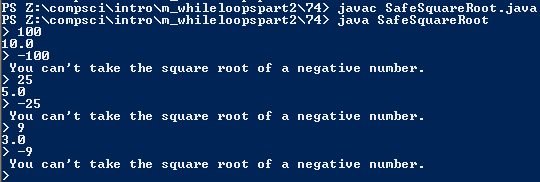# Assignemnt # and

## Code

```      ///Name: Dakota Donahue
///Period: 5
///Project Name:
///File Name:
///Date: 3/10/2016

import java.util.Scanner;

public class SafeSquareRoot
{
public static void main( String[] args )
{
Scanner keyboard = new Scanner(System.in);

double n, b;
int x = 100;

while ( x == 100 )
{
System.out.print("> ");
n  = keyboard.nextDouble();

while ( n > 0 )
{
b = Math.sqrt(n);

System.out.println(b);

System.out.print("> ");
n  = keyboard.nextDouble();
}

while ( n < 0 )
{
System.out.println( " You can't take the square root of a negative number. " );

System.out.print("> ");
n  = keyboard.nextDouble();
if ( n > 0 )
{
b = Math.sqrt(n);

System.out.println(b);
}
else
{
}
}
}
}
}

```